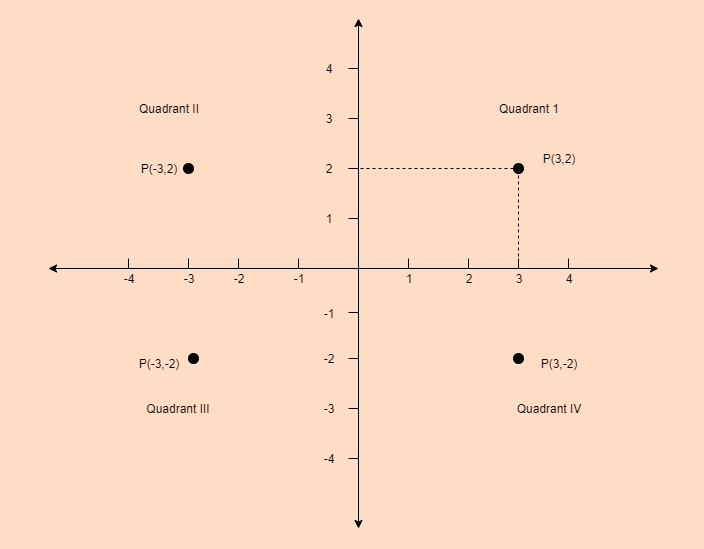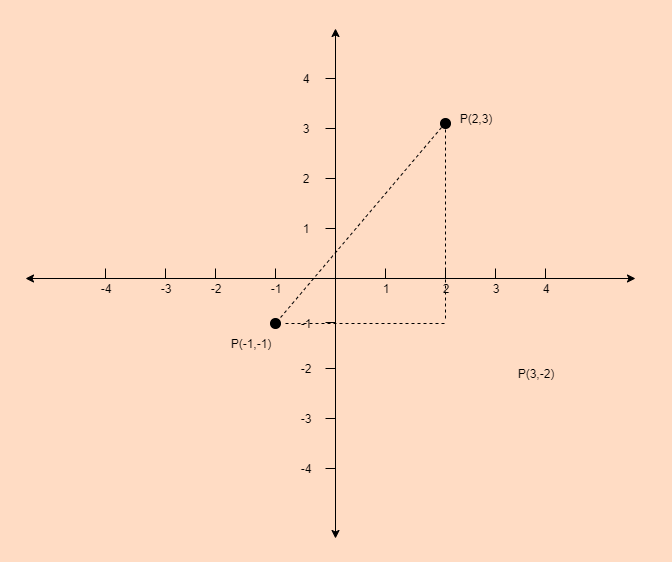# GRE Algebra | Coordinate Geometry

In the coordinate geometry, all the points are located on the coordinate plane. Coordinate plane is also called xy-plane.

The concepts of xy-plane are:

• The horizontal number line is called the x-axis and vertical number line is called the y-axis.
• The intersection point of both the axes is called the origin, denoted by O.
• The positive half of the x-axis right of the origin and negative half of x-axis is left to the origin.
• The positive half of the y-axis is above the origin and negative half of y-axis is below the origin.
• Two axes divide the plane into four Quadrants I, II, III and IV starting.In xy-plane every point is denoted by P(x, y). Here first number is called the x-coordinate and second number is called the y-coordinate. A point having coordinates (3, 2) is located 3 units to the right of y-axis and 2 units above to the x-axis as shown in the above plane.

Distance between two points:-
In xy-plane the distance between the two points (x1, y1) and (x2, y2) can be found by using the Pythagoras theorem.

Example: Find the distance between the points (2, 3) and (-1, -1).

Explanation:Distance between two points can be calculated using the below formula:

`Distance = √ [ (x2 - x1)2 + (y2 - y1)2) ] `

Put all the values,

```Distance
=  √[ (-1 - 2)2 + (-1 - 3)2) ]
=  √[ (-3)2 + (-4)2) ]
= √[ 9 + 16 ]
= √25
= 5 ```

Hence, distance between these two points is 5 unit.

My Personal Notes arrow_drop_upCheck out this Author's contributed articles.

If you like GeeksforGeeks and would like to contribute, you can also write an article using contribute.geeksforgeeks.org or mail your article to contribute@geeksforgeeks.org. See your article appearing on the GeeksforGeeks main page and help other Geeks.

Please Improve this article if you find anything incorrect by clicking on the "Improve Article" button below.

Article Tags :

Be the First to upvote.

Please write to us at contribute@geeksforgeeks.org to report any issue with the above content.